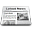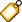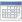#pROC 1.12.0

I just released pROC 1.12.0, which fixes several bugs and should significantly improve the performance on large datasets by selecting the best algorithm automatically.

## Issue 25

GitHub issue #25 identified two distinct bugs causing `ci.auc`, `var` and other functions to fail when calculating DeLong placements with the following error message, with different variation of numbers:

```Error in delongPlacements(roc) :
A problem occured while calculating DeLong's theta: got 0.50057161522129678399 instead of 0.50032663726931247972. This is a bug in pROC, please report it to the maintainer.```

pROC calculates the AUC with the trapezoidal method. This is the AUC obtained when calling `auc(roc)`. When using the DeLong method (for `roc.test`, `var` etc.), the AUC is also calculated with an other method (similar to the Wilcoxon/Mann-Whitney statistic). These two values should be identical, at least down to something close to the floating point precision of the hardware, typically below 10^-8. To be on the safe side, pROC checks this assumption after calculating the DeLong AUC.

The first sub-issue caused by a broken conversion of the roc curve from percent to fractions for internal calculations, followed by a broken check that the DeLong code produced the correct AUC. In combination, these two bugs caused pROC to stop with an error message in the specific case where `percent=TRUE` and `direction=">"`. The check was introduced in pROC version 1.9.1. The bug in the conversion from percent to fraction was present in earlier versions, however it never affected calculations, which is why it was left unnoticed until the check was added. Both bugs are now fixed.

The second sub-issue was impacting the calculation of the thresholds. When two predictor values were too close, their mean could not be represented exactly in the IEEE 754 arithmetic and the result would rounded back to one or the other value, pretty much arbitrarily depending on the implementation.

``` a <- c(0.65354946699793847742, 0.65354946699793858844)
> print(mean(a), digits = 20)
 0.65354946699793847742
> mean(a) == a
  TRUE FALSE```

Because pROC calculates the ROC thresholds as the mean between consecutive predictor observations, this would cause some comparisons to be incorrect when calculating sensitivity and specificity. As a consequence, erroneous sensitivities, sensitivities and AUCs may have been reported in the past. The issue was fixed by carefully selecting the correct value for threshold in case the mean was identical to a predictor value.

## Other bug fixes

• GitHub issue #27 caused `ci.auc` to return `NaN` when cases or controls contained only a single observation. The function has been fixed and now returns `NA` as expected.
• `power.roc.curve` failed with ROC curves having `percent=TRUE`. This issue was identified when adding testthat unit tests for the function.
• `ci(..., of="coords")` returned the `ci` function instead of calculating the CI.
• C++ code now check for user interrupts regularly with `Rcpp::checkUserInterrupt()` so that very long runs can be aborted from R.
• A better error message (instead of a useless internal garbage message error) is now displayed when attempting to return `threshold` with `ci.coords`. An empirical ROC curve, like one produced by pROC, is is made of discrete points that concentrate all the possible thresholds. Lines are added to join the points visually on the plot, however they do not contain any actual threshold. Returning thresholds at an arbitrary sensitivity or specificity requires either to be very lucky to have a point at the exact desired value, or to interpolate thresholds between the points. Interpolating is more tricky than it sounds and is very sensitive to the method of calculation of the threshold (very different results will be returned by pROC that uses the mean between consecutive predictor, than by some other packages which use the values directly).

## New algorithm

A new "meta" algorithm is introduced in the `roc` function. `algorithm = 5` causes pROC to automatically select algorithm 2 or 3, based on the number of threshold of the ROC curve. Algorithm 3 has a time complexity of O(N^2). It behaves very well when the number of thresholds remains low. However its square term can cause a catastrophic slowdown with very large datasets where the predictor takes nearly continuous values, and the ROC curve contains many thresholds (typically this will become very obvious above 10000 thresholds). Algorithm 2 has an algorithmic complexity of O(N), and shows a much better performance with large number of data points. However it comes with a rather large pre-factor which makes it very inefficient in most small- to normal-sized datasets. The decisive factor is the number of thresholds, and pROC will select algorithm 2 in curves with more than about 1500 thresholds, 3 otherwise. Algorithm 5 is now the default algorithm in pROC which should significantly improve the performance with large datasets, without impacting the speed in most cases.

## Getting the update

The update has just been submitted to the CRAN and should be online soon. Once it is out, update your installation by simply typing:

`install.packages("pROC")`

The full changelog is:

• Fix bug that crashed DeLong calculations when predictor had near-ties close to the floating point precision limit that were rounded back to a predictor value (issue #25).
• Fix bug that crashed `ci.auc` and `var` if `direction` was `">"` and `percent=TRUE` (issue #25).
• Fix bug causing `ci` to return `NaN` values with `method="delong"` when cases or controls had a single observation (issue #27).
• Fix `power.roc.curve` failed with curves having `percent=TRUE`.
• Fix `ci(..., of="coords")` returned the `ci` function instead of the CI.
• C++ code now check for user interrupts regularly with `Rcpp::checkUserInterrupt()`.
• Better error message for `ci.coords` attempting to return `threshold`.
• New algorithm = 5 (used by default) chooses the algorithm based on the number of thresholds to avoid worst case with algorithm = 3.

Xavier Robin
Publié le samedi 5 mai 2018 à 13:50 CEST
Lien permanent : /blog/2018/05/05/proc-1.12.0
Tags : pROC
Commentaires : 0

##Tags

Bruit de fond Hobbys Humour Informatique Internet Livres Logiciels Moi Mon site web Mozilla Photo Politique Programmation Scolaire Ubuntu pROC

##Calendrier

lun.mar.mer.jeu.ven.sam.dim.
123456
78910111213
14151617181920
21222324252627
28293031